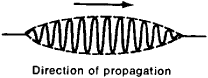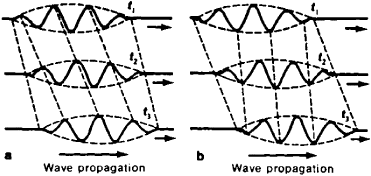# Group Velocity of Waves

The following article is from The Great Soviet Encyclopedia (1979). It might be outdated or ideologically biased.

## Group Velocity of Waves

the speed of movement of a group or train of waves; in the absence of absorption in the medium, it coincides with the rate of transfer of the energy of the wave group. An example of a wave group is the signal depicted in Figure 1.Figure 1. A wave group

A wave group is not a periodic wave (that is, it does not repeat exactly after a certain time interval) but rather consists of a set of harmonic waves whose frequencies lie within a certain interval. The narrower the interval, the smoother the form of the envelope of the wave group.

If the medium does not have dispersion, then all harmonic waves present in the group propagate at the same phase velocity. The envelope of the group also propagates at the same velocity; in this case the group velocity coincides with the phase velocity.

In the presence of dispersion, the harmonic waves of various frequencies that form the group are propagated at different phase velocities. Therefore, upon propagation, the relations among the phases of various harmonic waves change and distortion of the envelope takes place. However, if the phase velocities of a wave group differ little from one another (if the signal has a narrow spectrum), then the form of the envelope is maintained upon propagation and the influence of dispersion is manifested only in the fact that the rate of motion of the group envelope—that is, the group velocity of the waves—differs from the phase velocity.

Three successive instantaneous photographs of a narrow-spectrum signal propagating in a dispersive medium are shown in Figure 2. The slope of the dotted lines that connect points of identical phase (the maximums) characterizes the phase velocity, and the slope of the straight lines that connect the corresponding points of the envelope (the beginning and end of the signal) characterizes the group velocity of the signal. If upon propagation of the signal the maximums and minimums move more rapidly than the envelope, this means that the phase velocity of the given wave group exceeds its group velocity (Figure 2,a). Upon propagation of the signal, more and more new maximums, which are gradually displaced forward along the signal, reach its head, and disappear there. Such a situation may occur in the case of so-called normal dispersion—that is, in mediums where the phase velocity increases with increased frequency of the harmonic wave. Wave guides and substances transparent to optical waves are examples of mediums with normal dispersion. In a number of cases, however, anomalous dispersion of the medium is observed; in these cases the group velocity of the signal exceeds its phase velocity (Figure 2,b). The maximums and minimums appear in the forward part of the group, are displaced to the rear, and disappear in the tail of the signal. Anomalous dispersion is characteristic of waves on the surface of water and of light in absorbing mediums.Figure 2. Successive instantaneous views of a wave group at times t1, t2, and t3: (a) normal dispersion, (b) anomalous dispersion

The concept of group velocity plays a major role in a number of fields of physics, since any real harmonic wave, either electromagnetic or elastic, is actually a group of waves with close frequencies. Therefore, all methods of measuring the velocity of light in a substance that entail consideration of light lag produce group velocities. In the radio pulse sounding method, which is widely used to investigate the ionosphere, the delay times of signals reflected from the ionosphere are also determined by the group velocity of radio waves. In quantum mechanics the group velocity ∊ of waves is found to be equal to the velocity of the particle to which these waves are related.

### REFERENCES

Gorelik. G. S. Kolebaniio i volny, 2nd ed. Moscow, 1959.
Landsberg. G. S. Oplika, 4th ed. Moscow. 1957. (Obshchii kurs fiziki, vol. 3.)
Blokhintsev, D. I. Osnovy kvantovoi mekhaniki, 4th ed. Moscow, 1963.
The Great Soviet Encyclopedia, 3rd Edition (1970-1979). © 2010 The Gale Group, Inc. All rights reserved.
Site: Follow: Share:
Open / Close